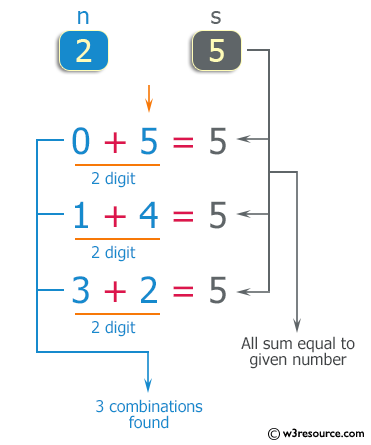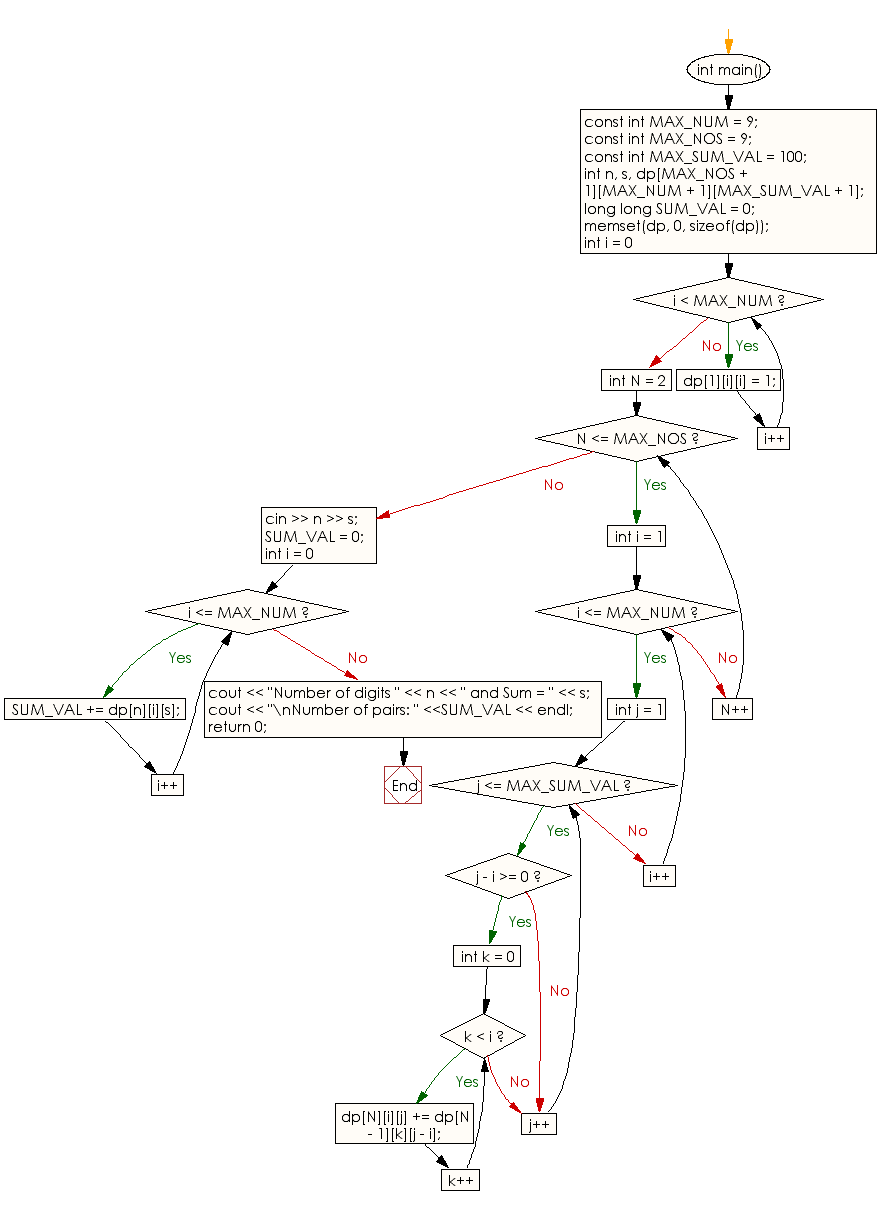﻿ C++ : Combinations, the sum of the digits equals a number

# C++ Exercises: Counts the number of combinations where the sum of the digits equals to given number

## C++ Basic: Exercise-72 with Solution

Write a C++ program that reads n digits chosen from 0 to 9 and counts the number of combinations where the sum of the digits equals the given number. Do not use the same digits in a combination.
For example, the combinations where n = 2 and s = 5 are as follows:
0 + 5 = 5
1 + 4 = 5
3 + 2 = 5

Pictorial Presentation:Sample Solution:

C++ Code :

``````#include <string.h>
#include <iostream>

using namespace std;

int main()
{
const int MAX_NUM  = 9;
const int MAX_NOS = 9;
const int MAX_SUM_VAL = 100;

int n, s, dp[MAX_NOS + 1][MAX_NUM + 1][MAX_SUM_VAL + 1];

long long SUM_VAL = 0;

memset(dp, 0, sizeof(dp));

for (int i = 0; i < MAX_NUM; i++)
{
dp[i][i] = 1;
}

for (int N = 2; N <= MAX_NOS; N++)
{
for (int i = 1; i <= MAX_NUM; i++)
{
for (int j = 1; j <= MAX_SUM_VAL; j++)
{
if (j - i >= 0)
{
for (int k = 0; k < i; k++)
{
dp[N][i][j] += dp[N - 1][k][j - i];
}
}
}
}
}

cin >> n >> s;

SUM_VAL = 0;

for (int i = 0; i <= MAX_NUM; i++)
{
SUM_VAL += dp[n][i][s];
}

cout << "Number of digits " << n << " and Sum = " << s;
cout << "\nNumber of pairs: " <<SUM_VAL << endl;

return 0;
}
``````

Sample Output:

```Sample Input: 2 5
Number of digits 2 and Sum = 5
Number of pairs: 3
```

Flowchart:C++ Code Editor:

What is the difficulty level of this exercise?

﻿

## C++ Programming: Tips of the Day

Why is there no std::stou?

The most pat answer would be that the C library has no corresponding "strtou", and the C++11 string functions are all just thinly veiled wrappers around the C library functions: The std::sto* functions mirror strto*, and the std::to_string functions use sprintf.

Ref: https://bit.ly/3wtz2qA

We are closing our Disqus commenting system for some maintenanace issues. You may write to us at reach[at]yahoo[dot]com or visit us at Facebook